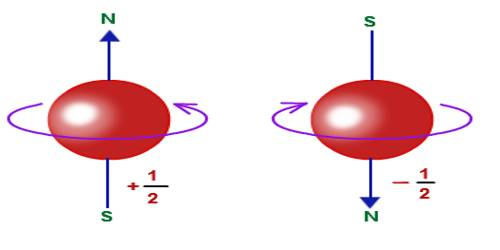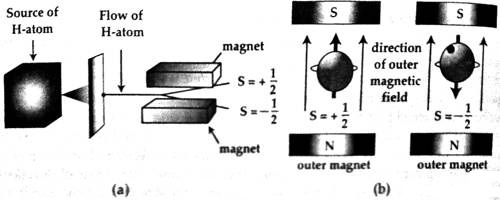Physics

# Electron Spin ExperimentElectron Spin Experiment

Electron spin is the electron’s electromagnetic field angular momentum. The electron spin is one of the three inherent properties of the electrons; the others are mass and charge of the electron. The electron spin is described as the spinning of the electron around its axis.

Due to the rotation of an electron in an orbit, intrinsic angular momentum is produced. It is the electron spin. Otto Stern and Walter Gerlach allowed a bunch of silver atoms to pass through a specially built magnetic field. Later on, the same experiment was performed with hydrogen atoms. It was seen that hydrogen atoms divided into two groups. Half of the atoms are deflected in one direction and the rest half of atoms are deflected in another direction [Fig. (a)]. As the particles are deflected by a magnet so the atoms also behave like a magnet.

The reason for the deflection of hydrogen atoms into two groups is that electron of each atom acts like a small magnetic dipole and they have two directions. Actually, the reason for this behavior of electrons is that each electron acts like a charged ball rotating in its own axis [Fig. (b)] and a charge while remains in rotation acquire magnetism; the two poles of that magnet depends on the direction of the rotation. Quantum conditions are applicable on electron spin and the values of spin can be either 1 only +1/2 or – 1/2.Again, the magnetic lines of force created by the equicentric orbits of atoms produce uniform magnetic field. The relation between the spin S and spin angular momentum μs can be expressed by the following equation.

μs = e/m S.

Here ‘e’ and ‘m’ are respectively the charge and mass of the electron.

The Spins plays a noteworthy role in quantum mechanics in computing the characteristics of elementary units like electrons. The direction of spin of the particle regulates several things like spin quantum number, angular momentum, the degree of freedom etc.